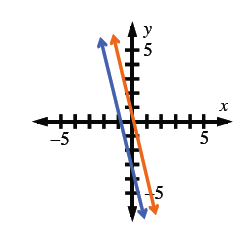### Home > AC > Chapter 4 > Lesson 4.2.4 > Problem4-106

4-106.

Graph the lines $y = −4x − 3$ and $y = −4x + 1$ on graph paper.

1. Where do they intersect?

2. Solve this system using the Equal Values Method.

3. Explain how your graph and algebraic solution relate to each other.

Try making tables if you are stuck.

Use the graph to complete the problems below.See problem 4-98.